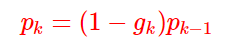# 0. 小结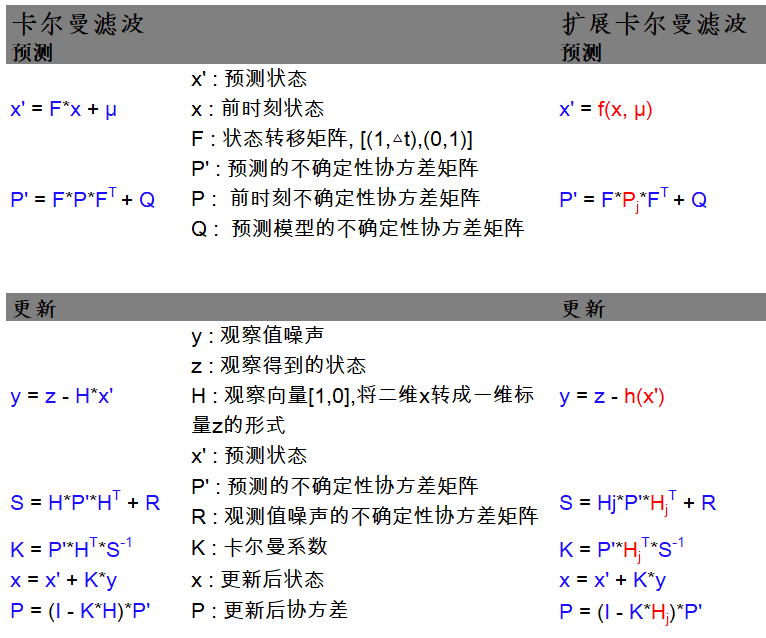# 1: A Simple Example

,如下图，这样可以根据时间差和之前的高度，来推定当前的高度：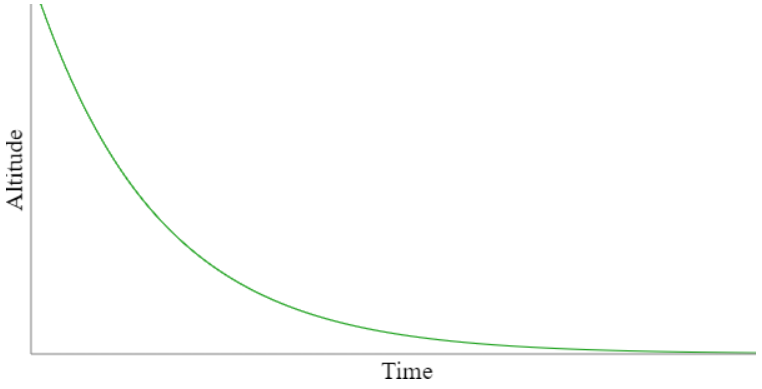# 2: Dealing with Noise

`observed_altitude(current_time) = altitude(current_time) + noise(current_time)`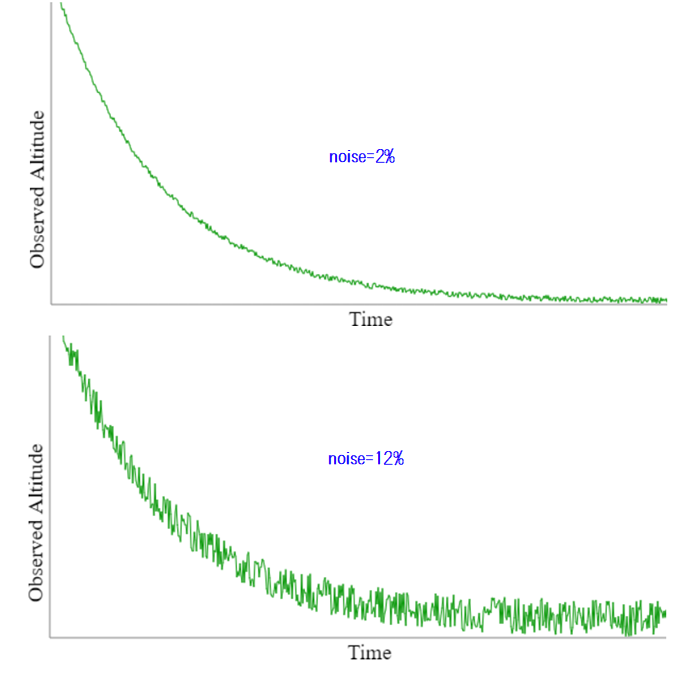# 3: Putting it Together

``````推测：altitude(current_time) = 0.95 * altitude(previous_time)

``````

``````x(k) = a * x(k-1)
z(k) = x(k) + v(k)
``````
1. x(k) 表示当前的系统状态，x(k-1)是上一个时间的系统状态
2. a表示某个常量
3. z(k) 表示当前的测量值，v(k)是当前的测量噪声

``````altitude(current_time) = 0.98 * altitude(previous_time) + turbulence(current_time)
``````

``````x(k) = a * x(k-1) + w(k)
``````

w(k)叫做过程噪声(process noise)，比如颠簸加减速等。

# 4: State Estimation

``````x(k) = a * x(k-1) + w(k)
x(k) = z(k) - v(k)    # 因为关注的是系统当前状态，所以把x(k)换到左边了
``````

Fortunately, Kalman had the insight that we can estimate the state by taking into account both the current observation and the previous estimated state. 通过当前的观测值和前时间段的估算值，来估计当前的状态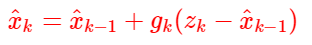g是一个gain参数，也叫卡尔曼系数，用于调节观测值和估算值，即看到底哪个值更可靠一些，举两个极端例子：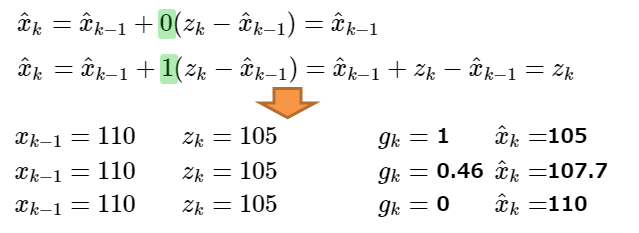# 5: Computing the Gain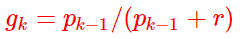p(k)是一个通过递归计算得到的预测误差：